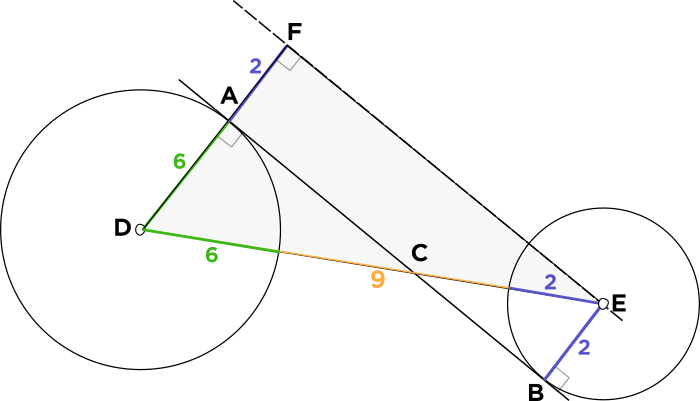# How can I solve this question if the distance between the 2 circles was not given?

• There was something similar in the weekly challenge. How can I solve this question if the distance between the 2 circles was not given?

• I don't think you can solve it without the distance between the two circles.

• @divinedolphin

The cool trick here is to visualize a large right triangle, $$\bigtriangleup FDE,$$ which you can get by sliding the tangent line $$\overline{AB}$$ over until it touches the center of the smaller circle:

First, it's helpful to remember that the question states that the circles are $$9$$ units apart at their closest point. This is equivalent to the yellow segment highlighted down below.Conveniently, the line connecting the two circles' centers, $$\overline{DE},$$ is just this segment of length $$9$$ plus the radius of $$6$$ and the radius of $$2,$$ so this line $$DE = 6 + 9 + 2 = 17.$$

Now we don't even need to know the Pythagorean Theorem, since we know of a right triangle with a leg of $$8$$ and a hypotenuse of $$17:$$ it's the $$8 - 15 - 17$$ right triangle! This means the other leg equal $$15,$$ which happens to be the length that we want!